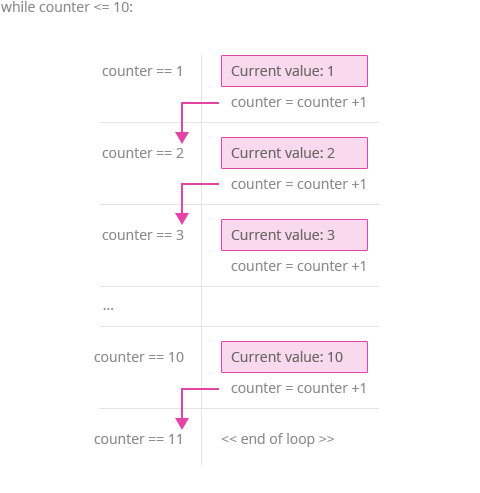Deals Of The Week - hours only!Up to 80% off on all courses and bundles.-Close
Introduction
The for loop
The while loop
8. while loop – explanation
Nested loops
break and continue
Summary

## Instruction

Good. Let's analyze what happened:

counter = 1
while counter <= 10:
print('Current value:', counter)
counter = counter + 1


The program above printed ten lines of text, each time with a different number: Current value: 1, Current value: 2, etc. We started the loop with the while keyword. After a space, we provided a condition (counter <= 10) that must hold true for the body of the loop to execute, followed by a colon (:). So long as the condition is true, the body of the loop will be executed repeatedly.

In our case, there is a variable named counter, whose initial value is 1. At the end of each iteration, we add 1 to the value of counter. After the final instruction in the loop code, we go back to the loop condition (counter <= 10). If it is still true, the whole loop is repeated in another iteration. After the iteration, the condition is checked again. Finally, after 10 iterations, counter equals 11 and the condition is no longer true, so the loop ends. Take a look at the picture:Remember, the body of the while loop has to be indented.

## Exercise

Modify the template code so that it only prints even numbers from 2 to 10.

### Stuck? Here's a hint!

Start the counter at 2, and add 2 each time at the end of the loop block.